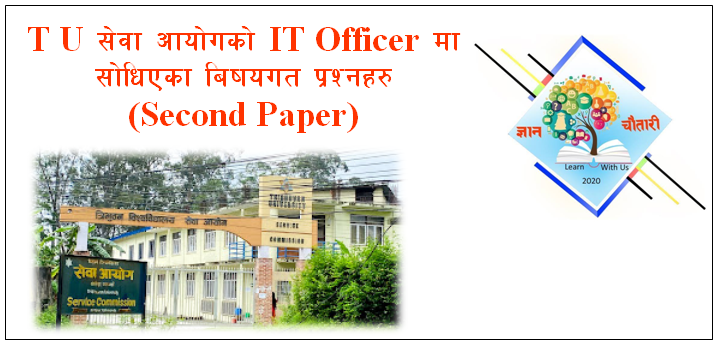# Question Paper of IT Officer of TU (Second Paper)

Tribhuvan University Service Commission

Open written Computational Examination (2078)

Applied post: Technical Officer (information and Technology)                Full Marks: 100

Paper: Second                                                                                                            Time: 3 hour

Candidates are required to give answers in their own words as far as practicable. All questions are compulsory. The figures in the margin indicate full marks.

1. Define DMA? What is the function of DMA address register? Explain various addressing modes present in 8086?                                                                          [1+2+7=10]
2. How do you implement the multiple inheritances in OOP? Explain with reference to oops programming code.                                                                           [4+6=10]
3. Why do we need to perform time and space analysis of algorithms? Distinguish between Depth-first searches (DFS) and Breadth-First Search (BFS) with appropriate example.                                                                                                                          [4+6=10]
4. Describe the database connection process in PHP and MySQL. How do you insert data after making connection? Explain with example.                                        [5+5=10]
5. What is concurrency control? Describe in details the various concurrency control technique.                                                                                                       [2+8=10]
6. Define Software testing. Explain various techniques used for testing software.[2+8=10]
7. What is deadlock? Describe with suitable example of the deadlock prevention methods.                                                                                                                          [2+8=10]
8. What do you mean by Domain Name System (DNS)? Explain in details how Domain Name System (DNS) Work?                                                                          [3+7=10]
9. What do you understand by cryptography? Explain in details the Public key infrastructure (PKI).                                                                                      [2+8=10]
10. Explain the Concept of Digital Nepal Framework.                                         5
11. Describe the roles and responsibilities of Executive Council of TU.              5